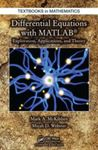## College of Arts & Sciences Faculty Books#### Title

Differential Equations with MATLAB: Exploration, Applications, and Theory

#### Description

A unique textbook for an undergraduate course on mathematical modeling, Differential Equations with MATLAB: Exploration, Applications, and Theory provides students with an understanding of the practical and theoretical aspects of mathematical models involving ordinary and partial differential equations (ODEs and PDEs). The text presents a unifying picture inherent to the study and analysis of more than 20 distinct models spanning disciplines such as physics, engineering, and finance.

9781466557079

7-10-2014

#### Publisher

Chapman and Hall/CRC Press

New York

#### Keywords

ordinary differential equations, partial differential equations, MATLAB

#### Disciplines

Partial Differential EquationsCOinS